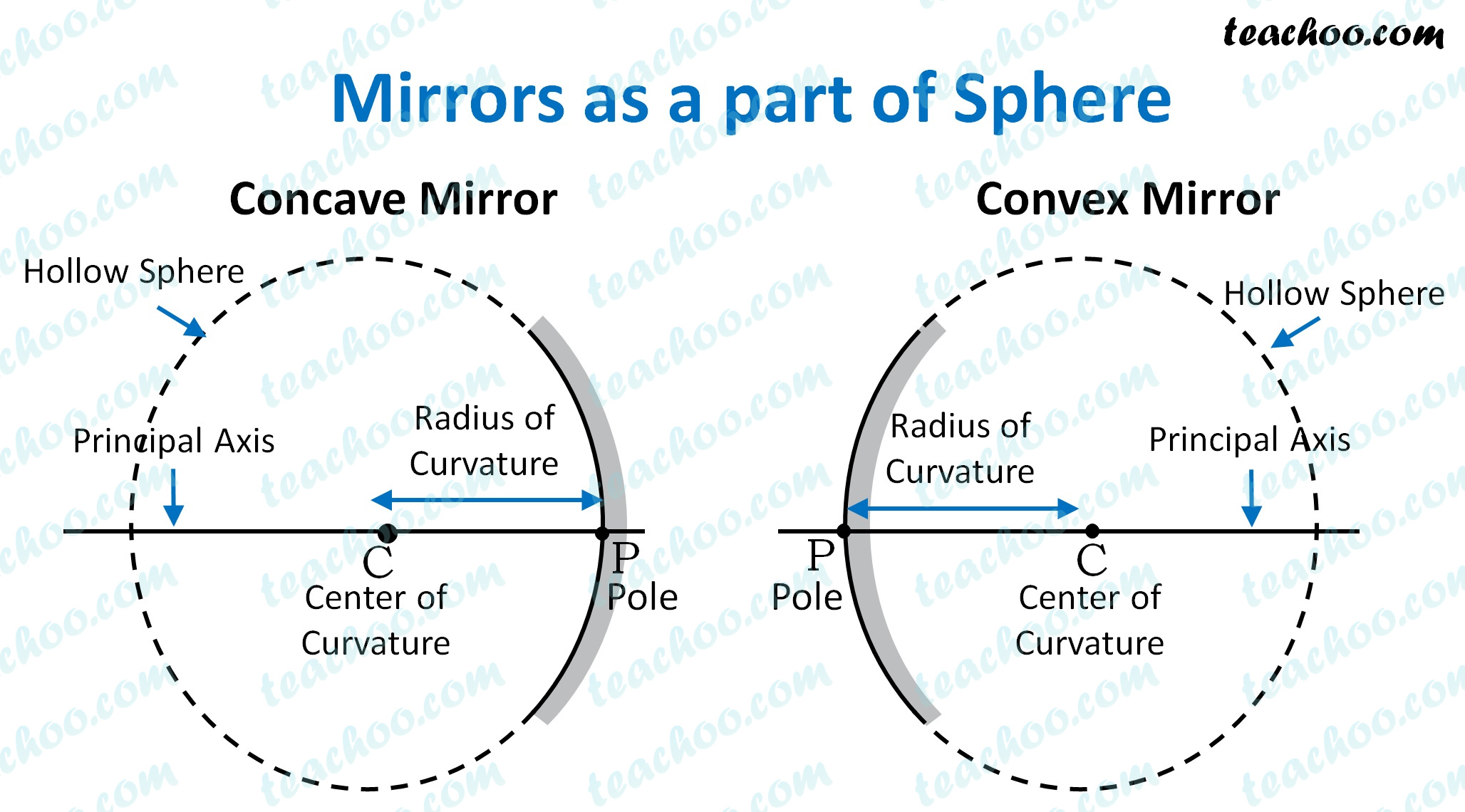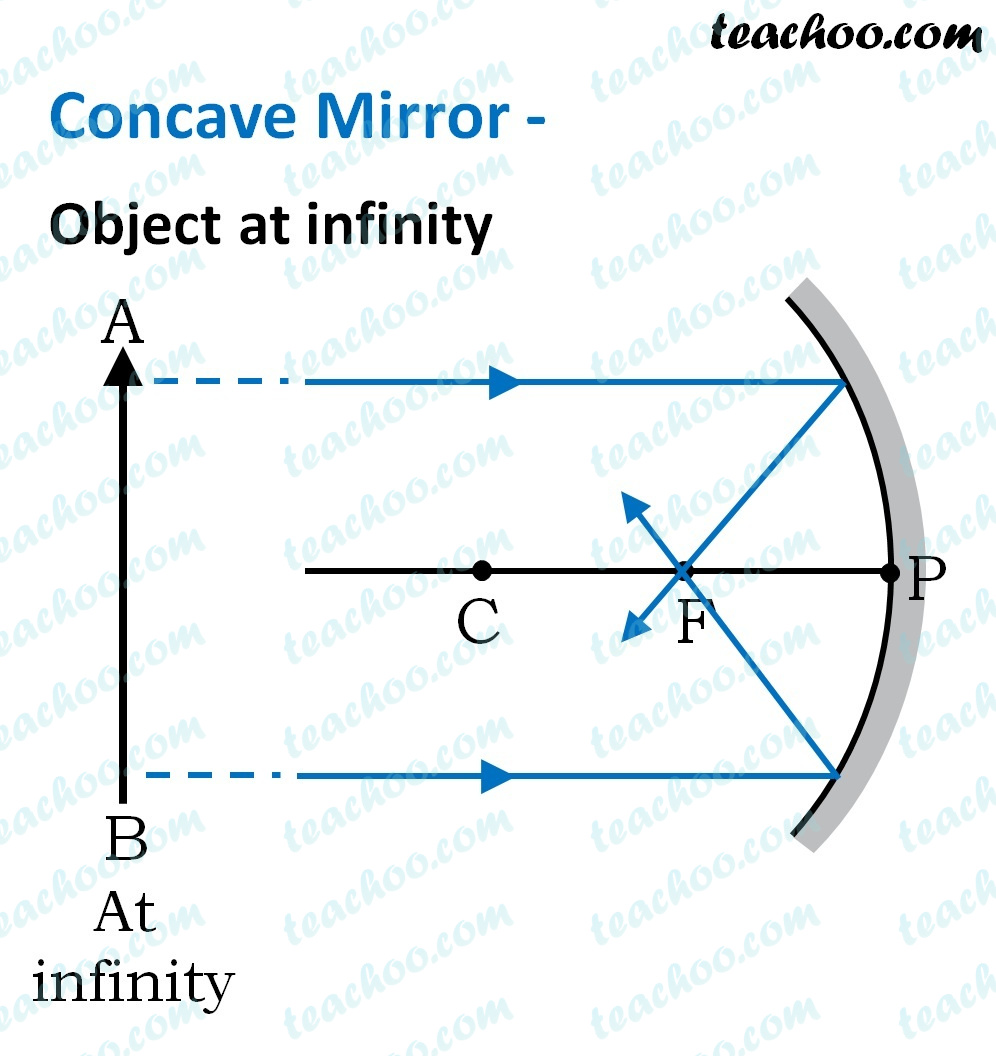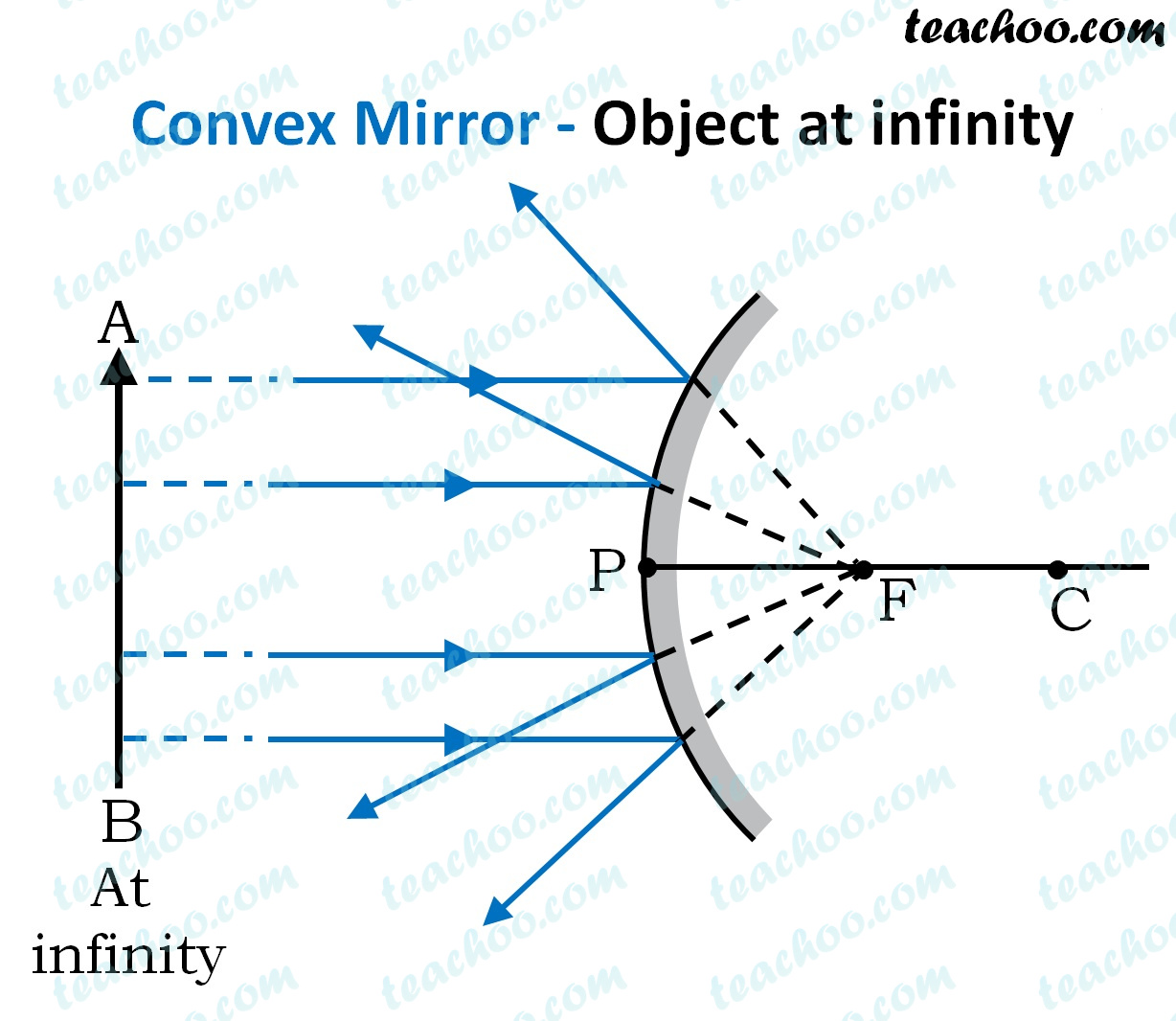Concepts

Class 10
Chapter 10 Class 10 - Light - Reflection and Refraction

We learned about Concave and Convex mirrors in the last post

But:

Did you know that Concave and Convex Mirrors are a part of a sphere?For these mirrors, we have some important terms like

• Center of Curvature
• Pole of the Mirror
• Principal Axis
• Focus of the mirror

Let's look at them one by one

## Center of Curvature (C)

We can say that the mirror is a part of the hollow sphere

The center of this sphere is the Center of Curvature

It is represented by the letter C

Note: - Center of curvature of Concave mirror is on the front, and of convex mirror is on the back

The radius of the hollow sphere which the mirror is a part of is the Radius of Curvature

It is represented by the letter R

Note : -  that R = PC

## Pole of a Mirror(P)

Center of the spherical mirror is called Pole

It is represented by the letter P

## Principal Axis

A straight horizontal line passing through pole and center of curvature

## Principal focus

For principal focus, let's look at Concave and Convex Mirrors separately

### Principal Focus (F) of concave mirror

The point at which

rays parallel to principal axis

coming from infinity converge

after being reflected from concave mirror

is called the principal focus of concave mirror.### Principal Focus (F) of convex mirror

The point at which

rays parallel to principal axis

coming from infinity appear to converge

after being reflected from convex mirror

is called the principal focus of convex mirror.Note: - Focus of convex mirror is behind the mirror, so it is a virtual focus

## Focal Length

It is distance between Pole (P) and Principal Focus (F)

It is represented by the letter f (small letter f)

## Relationship between Radius of Curvature and Focal Length Of Spherical Mirror

The focal length is equal to half of the radius of curvature, for any spherical mirror.

Hence

Focal length = Radius of curvature / 2

f = R/2

## Questions

Question 1 Page 168 - Define the principal focus of a concave mirror.

Question 2 Page 168 - The radius of curvature of a spherical mirror is 20 cm. What is its focal length?

Learn in your speed, with individual attention - Teachoo Maths 1-on-1 Class